# AP Chemistry : Reaction Types

## Example Questions

### Example Question #51 : Reaction Types

Which of the following can act as a Lewis base?

NH2NH2

CH3COOH

HF

BF3

NH2NH2

Explanation:

A Lewis base is an electron-pair donor. N atoms have a valence of 5, and in the NH2NH2 compound, it is only bonded to another N atom and 2 H, so it's only using 3 of its 5 valence electrons to form these bonds. Thus, each nitrogen has a pair of unbonded electrons and can act as a Lewis base.

### Example Question #42 : Acid Base Reactions

In the following equation, which is the conjugate base of HClO4?

HClO4 + H2O → ClO4– + H3O+

H3O+

ClO4–

HClO4

H2O

none of the above

ClO4–

Explanation:

The conjugate base of an acid will be the same compound, short one H atom. ClO4– is the only one that meets this criterion.

### Example Question #45 : Acid Base Reactions

Which of the following is a Lewis base?

CH3CH2CH2CH3

PH3

NH4+

CH2COOH

PH3

Explanation:

A Lewis base is an electron-pair donor. Only PH3 has a pair of nonbonding electrons and can act as a donor.

### Example Question #1 : Identifying And Defining Acids And Bases

Which of the following is the conjugate base of oxalic acid (H2C2O4)?

HC2O4+

HC2O4–

(C2O4) 2–

H2C2O4

HC2O4–

Explanation:

The conjugate base has one less H atoms and one unit greater negative charge because of this. Thus, the correct answer is HC2O4–

### Example Question #52 : Reaction Types

Which of the following solutions will have a pH greater than 7?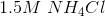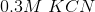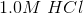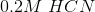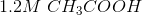Explanation:

HCN, HCl, CH3COOH, and NH4Cl are all acids (NH4+ is the ammonium ion). That only leaves KCN as the correct answer.

### Example Question #51 : Acid Base Reactions

BCl3 is a                     .

Lewis base

Bronsted-Lowry acid

Lewis acid

Arrhenius acid

Bronsted-Lowry base

Lewis acid

Explanation:

A Lewis acid is a species that can accept an electron pair. In the BCl3 molecule, B does not have a complete octet (3 covalent bonds, thus 6 electrons around it rather than 8). Thus, it can accept another electron pair, making it a Lewis acid.

### Example Question #51 : Acid Base Reactions

Which of the following compounds is a Lewis acid:,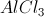,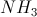,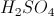?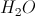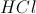Explanation:

A Lewis acid is a 2-electron acceptor, and a Lewis base is a 2-electron donor., while it is acidic, cannot accept two electrons, so it is not a Lewis acid.can accept two electrons, so it is a Lewis acid.is a Lewis base., like hydrochloric acid, is acidic, but cannot accept two electrons, so it is not a Lewis acid.

### Example Question #55 : Acid Base Reactions

Which of the following salts will result in an acidic solution?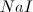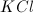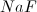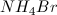Explanation:

All of the listed salts will dissolve into ions when in water. When the ions are in solution, they can act as acids or bases by donating or accepting protons. Chloride, bromide, and iodide ions are all conjugate bases of strong acids, so they will not accept protons. Sodium and potassium ions are the conjugate acids of strong bases, which dissociate completely, so they will not accept hydroxide ions.

Ammonium is the conjugate acid of ammonia, a weak base. The ammonium ion can donate a proton to the solution. This will make the solution slightly acidic. As a result, ammonium bromide is a salt that will make an acidic solution.

### Example Question #11 : Identifying And Defining Acids And Bases

Which of the following salts, when added to water, will result in a basic solution?

Ammonium bromide

Sodium fluoride

Potassium chloride

Sodium chloride

Sodium fluoride

Explanation:

A salt will dissociate completely in an aqueous solution, forming its respective ions. Knowing this, we can make predictions on how the ions will affect the pH of the solution, given their strengths as conjugate acids and bases. Fluoride ions are the conjugate base of hydrofluoric acid, which is a weak acid. As a result, fluoride ions will attach to protons in solution and decrease the proton concentration of the solution. This will make the solution more basic.

Chloride and bromide ions are conjugate bases of strong acids. Since these strong acids would dissociate completely in water, these ions will not associate with hydrogen ions in solution and will not affect the solution pH.

### Example Question #11 : Identifying And Defining Acids And Bases

Consider the following chemical reaction.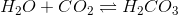What phenomena is this responsible for?

Dry ice

Acid rain

The violent reaction between baking soda and vinegar

When an acid and a base are mixed, a violent reaction occurs

Acid rain

Explanation:The above reaction describes the reaction that occurs when carbon dioxide dissolves in water and reacts to form carbonic acid, which is responsible for acid rain.

The phenomenon seen by dry ice is simply the sublimation of carbon dioxide. Acids and bases do not usually react in a violent fashion. The reaction between baking soda (sodium bicarbonate) and vinegar (acetic acid) is given below. The production of carbon dioxide gas is responsible for the bubbles seen in this reaction.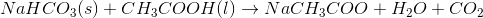### All AP Chemistry Resources